×#### Thank you for registering.

One of our academic counsellors will contact you within 1 working day.

Click to Chat

1800-1023-196

+91-120-4616500

CART 0

• 0

MY CART (5)

Use Coupon: CART20 and get 20% off on all online Study Material

ITEM
DETAILS
MRP
DISCOUNT
FINAL PRICE
Total Price: Rs.

There are no items in this cart.
Continue Shopping• Complete Physics Course - Class 11
• OFFERED PRICE: Rs. 2,968
• View Details

Magnetic Field and Lines of Force

Table of Content

Properties of a Magnet

Magnetic Field

Lines of Force – “Flux Lines”

Properties of Magnetic Lines of Force

Direction of magnetic lines of force –Physics

Representation of Magnetic Field

Related ResourcesA piece of substance which possesses the property of attracting small pieces of iron towards it is called a magnet.

If the property of magnesium occurs naturally the magnet is known as a natural magnet. It is possible, artificially, to induce magnetism by rubbing the given piece of substance with magnets. The magnet, thus produced, is called an artificial magnet.

Properties of a Magnet

A magnet possesses following properties:

(a) Two poles of a magnet

A magnet has two poles. One is ‘north seeking pole’ or simply north pole (N) while the second is ‘south seeking pole’ or the south pole (S). These poles are situated a little distance inside the faces of a magnet. Face to face length of the magnet is called geometric length while pole to pole length is called magnetic length of the magnet. It is quite evident that the magnetic length is slightly less than geometric length.

Magnetic length, 2l = (7/8)  geometric length

(b) Attracting property of a magnet

A magnet is capable of attracting small pieces of iron towards it. These pieces (iron filings) are attracted towards both the poles, north as well as south. This attraction is greatest at the poles and decreases as move towards the centre of magnet. It will be observed that the iron filings sticking to the magnet are largest in number near the poles and are smaller on the portion of magnet in between.

(c) Directional property of a magnet

When freely suspended a magnet always points in a particular direction. North pole of the magnet points towards geographic north pole while south pole points towards geographic south. That is the reason these poles are called north seeking pole ( or simply north pole) and south seeking pole (or simply south pole) respectively. The cause of this property is that earth behaves as a huge magnet which pulls the given magnet in a particular direction. This property led to the development of mariner’s compass which is extensively used for navigation purposes.

If the suspended magnet is deflected a bit from equilibrium position and released, it will execute torsional vibrations with N-S line as the mean position. Amplitude of vibrations will gradually decrease due to air resistance and the magnet will, ultimately, come to rest along N-S line.

(d) No existence of isolated magnetic poles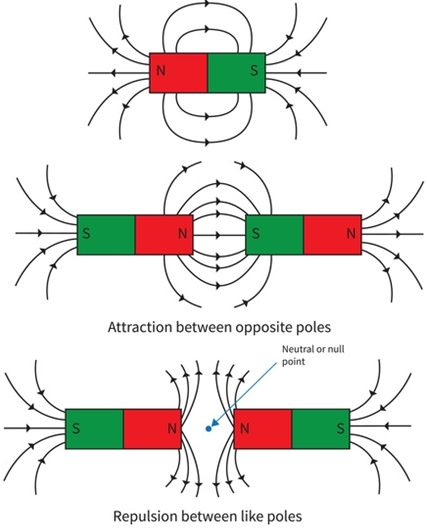The magnetic poles exist only in pairs of opposite nature. It is not possible to obtain an isolated magnetic, pole. If we break a magnet into two parts we get two independent complete magnets each with a pair of opposite pole. On further sub-division in to four pieces, we shall obtain four complete magnets again with a pair of opposite poles. This process continues till we reach the smallest particle i.e. an atom. The atom also behaves like a magnet. That is the reason we say that it is not possible to obtain an isolated pole. Sometimes, however, we need an isolated magnetic pole for theoretical consideration. In that case we can assume the pole of a very long magnet to be an isolated one, since the second pole will be situated at such a large distance away so as to be ineffective.

(e) Nature of force between two poles

Magnetic poles exert forces upon each other. The nature of force between similar pole is repulsive while that between opposite poles is attractive.

This can be observed from following simple experiment. Bring the north pole of a magnet near the north pole of a freely suspended magnet. The north of suspended magnet also rotates away. Similarly, it can be observed that south pole of suspended magnet also rotates away from the south pole, while the north pole of suspended magnet rotates towards the south pole of the magnet. This indicates that, “ like poles repel each other while unlike poles attract each other.”

(f) Magnitude of force between two poles

The magnitude of the force between two magnetic poles (supposed isolated) varies directly as the product of their pole and inversely as the square of the distance between them.

Consider two magnetic poles of similar nature (n) of strength m1 and m2 separated at a distance r from each other. The force (of repulsion) between them,

F ∝ m1m2

F∝ 1/r2   Or F ∝ m1m2/r2

Or   F = k [m1m2/r2]

Here, ‘k’ is the constant of proportionality.

In SI,  k =  µ0/4π

So, F = µ0/4π [m1m2/r2]

Here,  µ0 = 4π10-7 Wb A-1m-1

µ0 is called the “absolute magnetic permeability” of free space.

In C.G.S system, k = 1

Relation is termed as Coulomb’s law in magnetism.

Magnetic Field

Consider an isolated magnetic pole placed at any point. It experiences no force. When another magnetic pole is placed near it, a force starts acting on it. For the first pole, the properties of space around it have undergone a change due to placing of second pole near it. This space, with modified properties, is called the magnetic field of the second pole.

Magnetic field, of any magnetic pole, is the region (space) around it in which its magnetic influence can be realised.

The magnetic field which we plot, in laboratory, is actually a section of magnetic field in the horizontal plane (paper).

Refer this simulation for direction of magnetic field and current (Open right hand rule)

This illustrates the open right hand rule. We use it in conjunction with a demonstration/station. The demonstration has a wire traversing a powerful horseshoes magnet's open end. The wire is perpendicular to the horseshoe magnet's ends. When the end of the wire are attached to a battery the wire jumps.

Lines of Force – “Flux Lines”

Lines of force are the lines in any such field the tangent of which at any point gives the field direction at that point and its density gives the magnitude of the field. Hence, magnetic lines of force are basically the lines of force which represent the direction of the magnetic field. The imaginary path traced by an isolated (imaginary) unit north pole may also be defined as a line of force.

Magnetic lines of force are closed curves. Outside the magnet their direction is from north pole to south pole and inside the magnet these are from south to north pole.

Imagine a unit magnetic north pole to be situated, at any point, in a magnetic field. It experiences a force given by Coulomb’s law. If the north pole were completely free to move under the action of this force, it would move along a path called line of force.

Line of force is the path along which a unit north pole would move if it were free to do so.

In case of isolated magnetic poles the line of force is a straight line while in case of a combination of poles ( a magnet) it is curved line. The arrow head on the line indicates the direction of motion of the free north pole.

Properties of Magnetic Lines of Force

(a) The number of lines of force per unit area (area being perpendicular to lines) is proportional to magnitude of strength of field (magnetic intensity) at that point. Thus, more concentration of lines represents stronger magnetic field.

(b) The lines of force tend to contract longitudinally or lengthwise i.e. they possess longitudinal strain as shown in figure. Due to this property the two unlike magnetic poles attract each other.

(c) The lines of force tend to exert lateral (sideways) pressure, i.e., they repel each other laterally. This explains the repulsion between two similar magnetic poles.

(d) 4π lines of force start from a unit magnetic pole.

Watch this Video for more reference

Direction of magnetic lines of force –Physics

The direction of magnetic lines of force at any point gives the direction of the magnetic force on a north pole placed at that point.

Magnetic Lines of force emerge from North Pole. Why?

The direction of magnetic line of force is the direction of force on a North Pole, so the magnetic lines of force always begin on the North Pole of a magnet and end on the South Pole of the magnet. When a small magnetic compass is placed along a lie of force, it sets itself along the line tangential to it. Hence, the line drawn from the South Pole of the compass to its North pole shows the direction of the magnetic field.

Do magnetic lines of force never cross each other? Explain. Does the direction of the magnetic field change at any point along the magnetic lines of force?

It is not so that the magnetic field lines cannot cross but whenever such a situation arises, the field strength has to be zero. A magnetic field line is the path that points in the direction of the magnetic field at every point along it. If one moves with a compass along a magnetic field line, we will notice that the needle always points in the direction the person wants to head in, in order to stay on that magnetic field. If two field lines cross, then it would mean that the magnetic field points in two different directions at one place. But since there is only of the magnetic field at any place at any moment, hence this does not happen.

Representation of Magnetic Field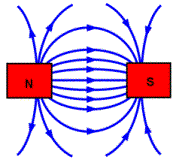A magnetic field can be represented by a set of magnetic lines of force. If the lines are spaced widely apart, it is a weak field. If the lines are situated close to each other, it represents a strong field. A magnetic field is of two types:

(a) Uniform field

A magnetic field is said to be uniform if it has same strength (in magnitude and direction) at all the points. It is represented by a set of parallel lines of force.

(b) Non-uniform field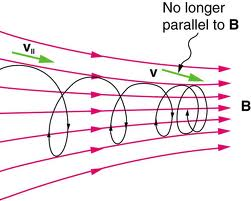A magnetic field is said to be non-uniform if it has different field strength at different points. It is represented by a set of convergent or divergent lines of force.

(c) Varying Magnetic Field:

The magnetic field which keeps on changing with respect to time is called as a variable magnetic field.

Example: B = B0 sin ωt

B = B0 cos ωt

(d) Non-varying Magnetic Field:

The magnetic field which does not change with time is called as a constant magnetic field. The direction of magnetic field is that in which a force acts on a unit test pole. It can be produced by moving charges, current carrying loops, and variations in electric currents.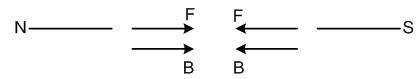Lines of force are directed away from a north pole and are directed towards a south pole. A line of force starts from a north pole and ends at a south pole if they are isolated poles.

Tangent, at any point, to the magnetic line of force gives the direction of magnetic intensity at that point.

Two lines of force never cross each other. If the two lines were to cross, two tangents could be drawn to the lines of force at the common point meaning thereby two directions of magnetic intensity at that point, which is obviously not possible.

The lines of force do not exist actually, but are imaginary lines that are used to illustrate and describe the pattern of the magnetic field.

The lines of force travel inside the magnet from the South Pole to the North Pole and hence complete the loop.

The lines of force don’t have any origin or end and do not interact because if they do so then it would mean two value of magnetic field at a single point, which is not possible.

The number of magnetic lines of force passing through unit normal area is defined as magnetic induction whereas the number of lines of force passing through any area is known as magnetic flux.

Two thin long parallel wires separated by a distance b are carrying a current i ampere each. The magnitude of the force per unit length exerted by one wire on the other is

(a) µ0i2/b2         (b) µ0i2/2πb

(c) µ0i/2πb       (d) µ0i/2πb2

Solution:

Force per unit length between two wires carrying currents i1 and i2 at distance r is given by

F/L = (µ0/2π) (i1i2/r)

Here, i1 = i2 = i and r = b

Thus, F/L =  µ0i2/2πb

Therefore, from the above observation we conclude that, option (b) is correct.Question 1

A magnetic field line is used to find the direction of

(a) south – north             (b) a bar magnet

(c) a compass needle      (d) magnetic field

Question 2

An electric current passes through a straight wire in the direction of south to north. Magnetic compasses are placed at points A and B as shown in the figure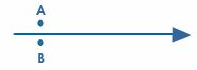(a) The needle will not deflect

(b) Only one of the needles will deflect

(c) Both the needles will deflect in the same direction

(d) The needles will deflect in the opposite directions

Question 3

The two poles in a magnet have

(a) equal pole strength

(b) unequal pole strength

(c) can be same or different

(d) None of these

Question 4

Magnetic field lines:

(a) from closed curves

(b) cannot intersect

(c) are crowded near poles

(d) All of these

Question 5

The magnetic field produced due to the current passing through a conductor is proportional to the _______.

(a) electric current            (b) conducting material

(c) length of conductor      (d) diameter of conductorQ.1
Q.2
Q.3
Q.4
Q.5

d

d

a

d

a

Related Resources

You might like to magnetic moment.

For getting an idea of the type of questions asked, refer the  Previous Year Question Papers.### Course Features

• 101 Video Lectures
• Revision Notes
• Previous Year Papers
• Mind Map
• Study Planner
• NCERT Solutions
• Discussion Forum
• Test paper with Video Solution